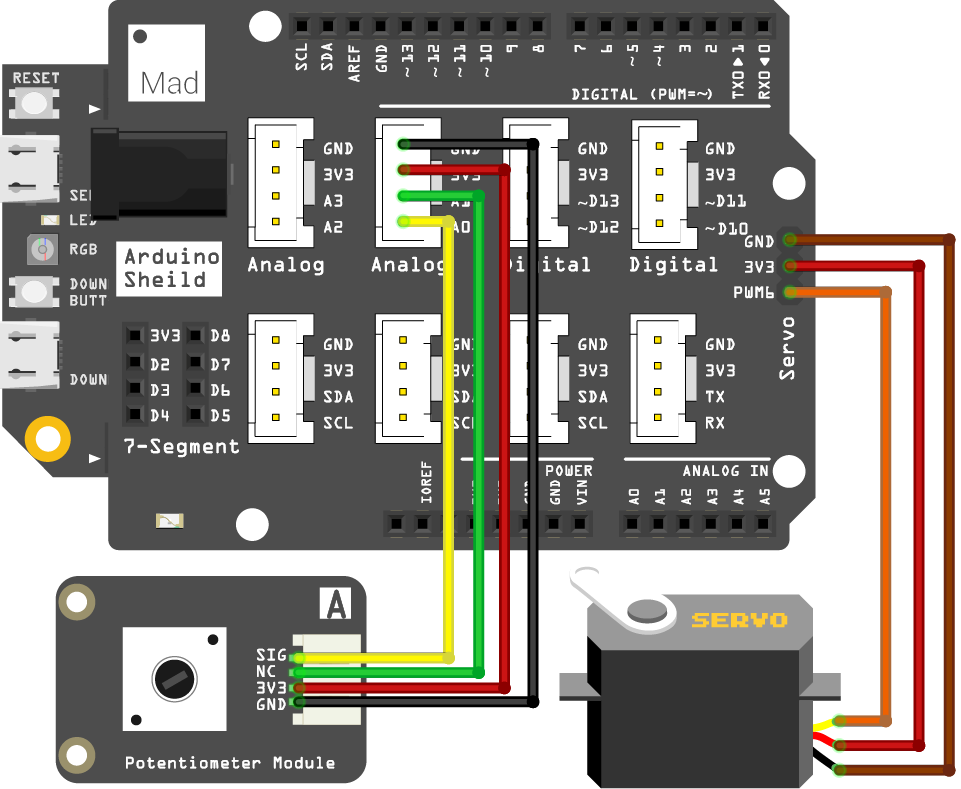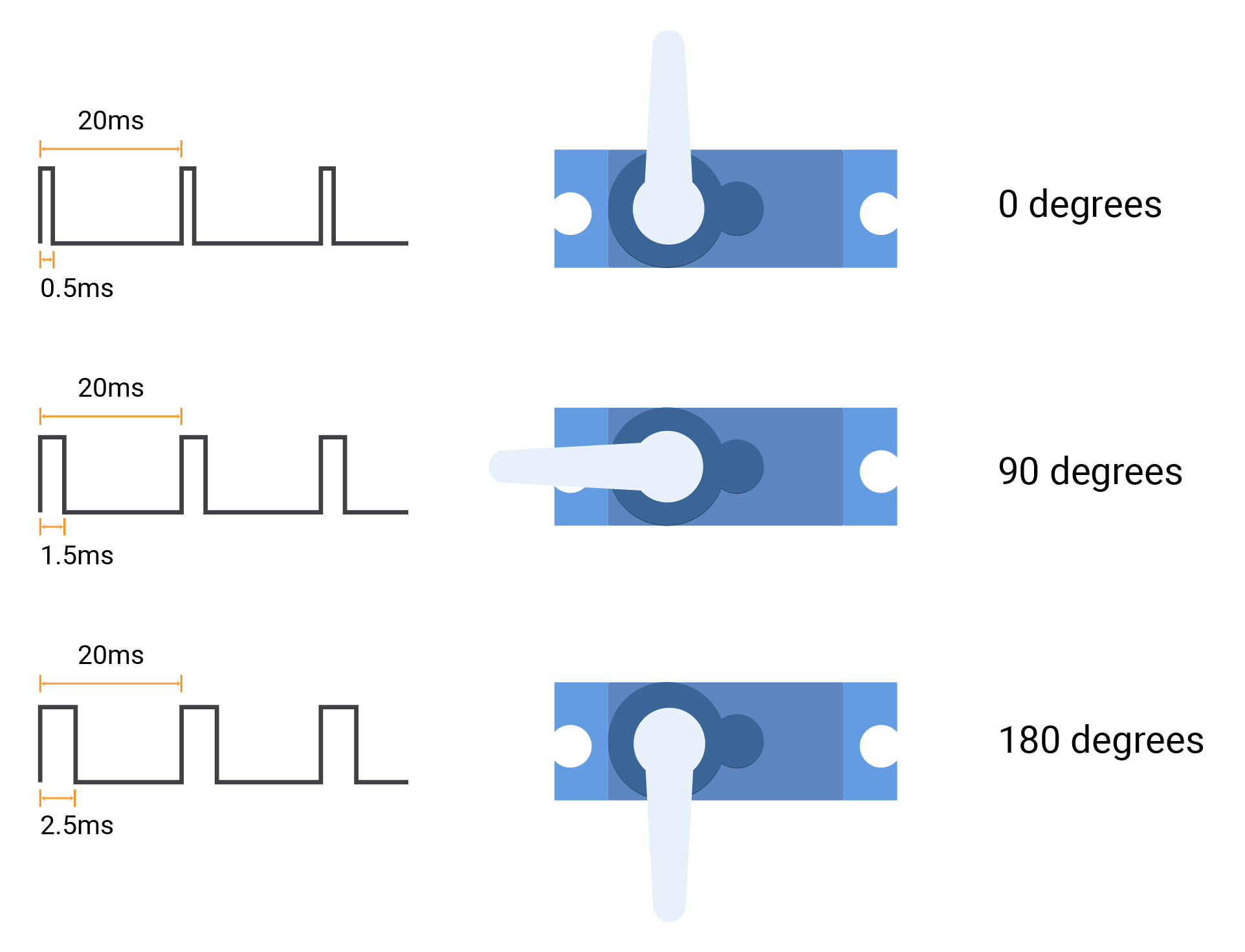# Mission8_Servo_Motor

In this mission, you will use another kind of motor, the servo motor. The angle of its arm will change with the potentiometer.

## What you need​

The parts you will need are all included in the Maker kit.

• SwiftIO board
• Shield
• Servo
• Potentiometer module
• 4-pin cable

## Circuit​

1. Place the shield on top of your SwiftIO board.

2. Connect the potentiometer module to pin A0 using a 4-pin cable.

3. Connect the servo to the pin PWM4A. The servo has three wires: the ground wire is brown, the power wire is red, and the signal wire is orange.## Example code​

``// Import the SwiftIO library to use everything in it.import SwiftIO// Import the board library to use the Id of the specific board.import MadBoard// Initialize the analog pin and PWM pin.let a0 = AnalogIn(Id.A0) let servo = PWMOut(Id.PWM4A)while true {    // Read the analog value and return a value between 0.0 and 1.0.    let value = a0.readPercent()     // Calculate the value to get the pulse duration.    let pulse = Int(500 + 2000 * value)     // Set the servo position according to the pulse.    servo.set(period: 20000, pulse: pulse)     sleep(ms: 20)}``

## Background​

### Servo motor​

The servo motor can adjust its arm's position according to the signal. You can set PWM output to control it. This servo requires a pulse every 20ms. And the duration of each pulse determines the position of the arm:• If the high voltage lasts for 0.5ms, the arm will be at 0 degrees.
• If the high voltage lasts for 1.5ms, the arm will be at 90 degrees.
• If the high voltage lasts for 2.5ms, the arm will be at 180 degrees.
##### note

This servo needs the pulse to be in the range of 0.5 to 2.5ms. You may also meet other servos that need a pulse in 1 to 2ms.

## Code analysis​

``import SwiftIOimport MadBoard``

Import the two libraries: SwiftIO and SwiftIOBoard. SwiftIO is used to control the input and output of the SwiftIO board. SwiftIOBoard defines the pin name of the board.

``let a0 = AnalogIn(Id.A0)let servo = PWMOut(Id.PWM4A)``

Initialize the analog pin A0 for the potentiometer and the PWM pin PWM4A for the motor.

``let value = a0.readPercent() let pulse = Int(500 + 2000 * value)servo.set(period: 20000, pulse: pulse)sleep(ms: 20)``

In the dead loop, read the input value in percentage, so you get a value between 0 and 1.

You will use this method `set(period:pulse:)` to set the PWM. The period is in microseconds, so it is 20000us. The pulse should be the number between 0.5ms to 2.5ms. So the value will be matched into this range by doing some calculations.

## Reference​

PWMOut - set the PWM signal.